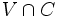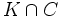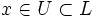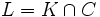# Local compactness is weakly hereditary

(diff) ← Older revision | Latest revision (diff) | Newer revision → (diff)
This article gives the statement, and possibly proof, of a topological space property satisfying a topological space metaproperty
View all topological space metaproperty satisfactions | View all topological space metaproperty dissatisfactions
|
Property "Page" (as page type) with input value "{{{property}}}" contains invalid characters or is incomplete and therefore can cause unexpected results during a query or annotation process.
Property "Page" (as page type) with input value "{{{metaproperty}}}" contains invalid characters or is incomplete and therefore can cause unexpected results during a query or annotation process.

## Statement

### Property-theoretic statement

The property of topological spaces of being locally compact satisfies the metaproperty of topological spaces of being weakly hereditary.

### Verbal statement

Any closed subset of a locally compact space is locally compact.

## Proof

Given: A locally compact space$X$, a closed subset$C$

To prove:$C$ is locally compact

Proof: We need to show that given any point$x \in C$, there exists an open subset containing$x$ contained in a closed compact subset of$C$.

Since$X$ is locally compact, there exists an open set$V \ni x$ and a closed compact subset$K$ of$X$ containing$V$.

By the definition of subspace topology,$V \cap C$ is an open subset of$C$. Call this$U$. Further,$K \cap C$ is a closed subset of$C$. Call this$L$. We then have$x \in U \subset L$, with$U$ open and$L$ closed.

We need to show that$L$ is compact. For this, observe that$L = K \cap C$, and$C$ is closed in$X$, so$L \subset K$ is closed as a subset of$K$. Since any closed subset of a compact space is compact, we conclude that$L$ is compact, completing the proof.